MATLAB Filter Tool

Ryan McGee

RMM052000@utdallas.edu

MATLAB files: FD.m
Manual: FD_Manual.doc

ABSTRACT

The goal of this project was to create a MATLAB program to automate the design of notch filters, resonators, and oscillators.  Based on user input of desired parameters, MATLAB will generate the appropriate linear constant coefficient difference equation (LCCDE).  The LCCDE of each filter is output to the user along with a graph of the respective frequency response or waveform.

PROCEDURE

Setting the Sampling Frequency

For each filter the user is able to specify the sampling frequency, FS, and, F0, the frequency to be notched in the case of FIR or IIR notch filters, or resonated in the case of all-pole or pole-zero resonators.  For oscillators, the user can set the sampling and oscillating frequency.  The user will be warned if FS does not exceed twice F0, for this does not satisfy the Nyquist theorem and will result in an inaccurate response.

Setting the Gain

Additionally for the notch filters and resonators, the user is allowed to specify the gain in dB at a particular frequency of the filter (FG).  FG is normalized by the sampling frequency and converted to radians to obtain w0.    The default gain at FG of each filter is computed and then normalized to obtain the user’s desired gain at FG.

Obtaining the Impulse Response

To derive an impulse response for a filter, one must first plot the zeros of the filter on the unit circle (r = 1) in the complex z-plane.  The resulting z-domain equation can be easily converted to the discrete vector and made causal by multiplying by a power of z.  The IIR notch, all-pole, and pole-zero resonator all have poles lying at the same angle as their zeros but at distance of r < 1 from the origin.  Values of r closer to 1 (closer to each zero) will result in a narrower notch or resonator.

The derivations for each filter were done by hand and vectors containing the n-domain values were entered into MATLAB.  Also, the user is prompted to enter a value of r for each filter and given restrictions (0 ≤ r < 1).

Generating the LCCDE

I derived by hand the LCCDE from each filter’s impulse response by converting from the z-domain to the discrete domain (n-domain).  I input the standard form of each LCCDE into MATLAB as a string that calls upon my impulse response vectors to obtain the proper coefficients.  The result is a neat printout of each particular filter’s LCCDE based on the user’s specified parameters.

The Oscillator

Specifying the gain of the oscillator is irrelevant in this case because the oscillator is a waveform generator rather than a filter.  The user is simply asked for the sampling frequency and oscillating frequency.  My program generates the appropriate waveform from the LCCDE of the oscillator.

EXAMPLES OF RESULTS

FIR Notch

 Choose one of the following or type 999 to quit.  1. FIR Notch  2. IIR Notch  3. All-Pole Resonator  4. Pole-Zero Resonator  5. Oscillator  1 Sampling Frequency: 400 Notch Frequency: 80 Frequency to Set Gain: 0 Gain (dB) at 0Hz: 0   LCCDE =   y(n) = 0.7236[1x(n) + -0.618x(n-1) + 1x(n-2)]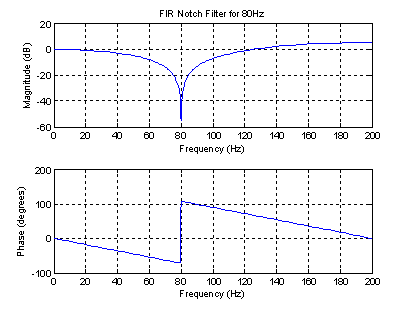IIR Notch

 Choose one of the following or type 999 to quit.  1. FIR Notch  2. IIR Notch  3. All-Pole Resonator  4. Pole-Zero Resonator  5. Oscillator  2 Sampling Frequency: 600 Notch Frequency: 60 r: .98 Frequency to Set Gain: 20 Gain (dB) at 20Hz: 10   LCCDE =   y(n) + -1.618y(n-1) + 0.9604y(n-2) = 2.801[x(n) + 1x(n-1) + x(n-2)]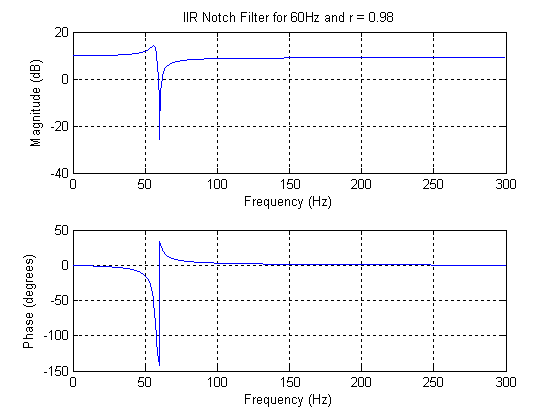All-Pole Resonator

 Choose one of the following or type 999 to quit.  1. FIR Notch  2. IIR Notch  3. All-Pole Resonator  4. Pole-Zero Resonator  5. Oscillator  3 Sampling Frequency: 250 Resonating Frequency: 100 r: .95 Frequency to Set Gain: 0 Gain (dB) at 0Hz: 10   LCCDE =   y(n) + 1.537y(n-1) + 0.9025y(n-2) = 10.88x(n)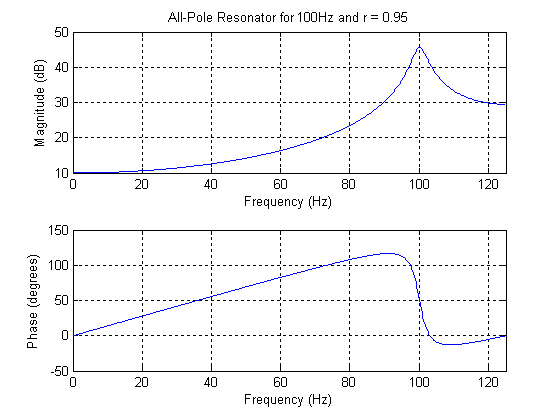Pole-Zero Resonator

 Choose one of the following or type 999 to quit.  1. FIR Notch  2. IIR Notch  3. All-Pole Resonator  4. Pole-Zero Resonator  5. Oscillator  4 Sampling Frequency: 900 Resonating Frequency: 70 r: .99 Frequency to Set Gain: 10 Gain (dB) at 10Hz: 5   LCCDE =   y(n) + -1.748y(n-1) + 0.9801y(n-2) = 2.894[x(n) - x(n-2)]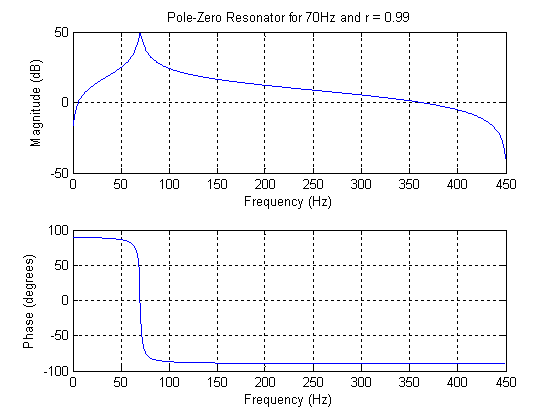Oscillator

 Choose one of the following or type 999 to quit.  1. FIR Notch  2. IIR Notch  3. All-Pole Resonator  4. Pole-Zero Resonator  5. Oscillator  5 Sampling Frequency: 500 Oscillating Frequency: 12   LCCDE =   y(n) + -1.977y(n-1) + y(n-2) = 0.1502x(n-1)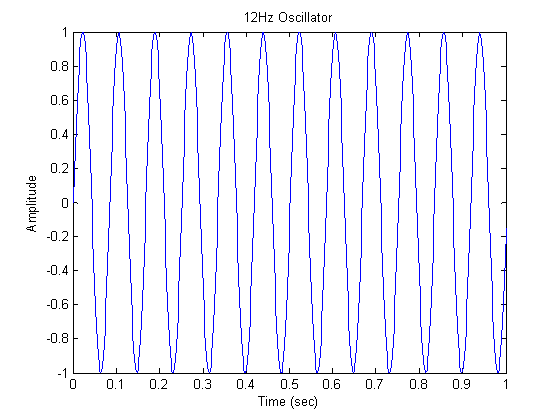CONCLUSION

While the theory behind construction of digital filters can take some time to fully understand, it is no more difficult than the theory behind analog filters.  However, the main difference between analog and digital lies in the ease of implementation.  Whereas analog circuits must be physically constructed with parts lacking perfect tolerance, digital filters and oscillators can be more precisely constructed using relatively few lines of code and with no cost for parts (besides a computer).
MATLAB CODE

 %Filter Designer (FD.m)   choice = input('Choose one of the following or type 999 to quit.\n 1. FIR Notch \n 2. IIR Notch \n 3. All-Pole Resonator \n 4. Pole-Zero Resonator \n 5. Oscillator \n ');   if(choice == 1)       %FIR notch filter       FS = input('Sampling Frequency: ');     F0 = input('Notch Frequency: ');     if (F0 > FS/2)         warnstr =['WARNING! The Sampling frequency is less than twice the notch frequency. Response will be inaccurate.']     end     FG = input('Frequency to Set Gain: ');     if (FG == F0)         warnstr =['WARNING! User should not set the gain of the notch frequency. Response will be inaccurate.']     end     fgstr = ['Gain (dB) at ' num2str(FG) 'Hz: '];     FGAINdB = input(fgstr);     FGAIN = 10^(FGAINdB/20);         w0 = 2*pi*F0/FS;     wg = 2*pi*FG/FS;         GH = abs(1 - 2*cos(w0)*exp(-j*wg) + exp(-j*2*wg));     G = FGAIN/GH;         h = [1 -2*cos(w0) 1];         freqz(G*h,1,[], FS);         titlestr = ['FIR Notch Filter for ' num2str(F0) 'Hz'];     title(titlestr)         LCCDE = ['y(n) = ' num2str(G, 4) '[' num2str(h(1), 4) 'x(n) + ' num2str(h(2), 4) 'x(n-1) + ' num2str(h(3), 4) 'x(n-2)]']   elseif(choice == 2)       %IIR Notch       FS = input('Sampling Frequency: ');     F0 = input('Notch Frequency: ');     if (F0 > FS/2)         warnstr =['WARNING! The Sampling frequency is less than twice the notch frequency. Response will be inaccurate.']     end     r = input('r: ');     if (r >= 1 || r < 0)         warnstr =['WARNING! r should be a positive value < 1']     end     FG = input('Frequency to Set Gain: ');     if (FG == F0)         warnstr =['WARNING! User should not set the gain of the notch frequency. Response will be inaccurate.']     end     fgstr = ['Gain (dB) at ' num2str(FG) 'Hz: '];     FGAINdB = input(fgstr);     FGAIN = 10^(FGAINdB/20);         w0 = 2*pi*F0/FS;     wg = 2*pi*FG/FS;         bg = abs(1 - 2*cos(w0)*exp(-j*wg) + exp(-j*2*wg));     ag = abs(1 - 2*cos(w0)*exp(-j*wg) + (r^2)*exp(-j*2*wg));     GH = bg/ag;     G = FGAIN/GH;         b = [1 -2*cos(w0) 1];     a = [1 -2*cos(w0) (r^2)];         freqz(G*b,a,1024, FS);     titlestr = ['IIR Notch Filter for ' num2str(F0) 'Hz and r = ' num2str(r)];     title(titlestr)         LCCDE = ['y(n) + ' num2str(b(2), 4) 'y(n-1) + ' num2str((r^2), 4) 'y(n-2) = ' num2str(G, 4) '[x(n) + ' num2str(b(1), 4) 'x(n-1) + x(n-2)]']   elseif(choice == 3)       %All-Pole Resonator       FS = input('Sampling Frequency: ');     F0 = input('Resonating Frequency: ');     if (F0 > FS/2)         warnstr =['WARNING! The Sampling frequency is less than twice the resonating frequency. Response will be inaccurate.']     end     r = input('r: ');     if (r >= 1 || r < 0)         warnstr =['WARNING! r should be a positive value < 1']     end     FG = input('Frequency to Set Gain: ');     fgstr = ['Gain (dB) at ' num2str(FG) 'Hz: '];     FGAINdB = input(fgstr);     FGAIN = 10^(FGAINdB/20);         wr = 2*pi*F0/FS;     if (r >= 0.9)         w0 = wr;     else         w0 = acos((2*r*cos(wr))/(1 + r^2));     end         wg = 2*pi*FG/FS;         ag = abs(1 - 2*r*cos(w0)*exp(-j*wg) + (r^2)*exp(-j*2*wg));     GH = 1/ag;     G = FGAIN/GH;         a = [1 -2*r*cos(w0) (r^2)];       freqz(G,a,[], FS);     titlestr = ['All-Pole Resonator for ' num2str(F0) 'Hz and r = ' num2str(r)];     title(titlestr)       LCCDE = ['y(n) + ' num2str(a(2), 4) 'y(n-1) + ' num2str((r^2), 4) 'y(n-2) = ' num2str(G, 4) 'x(n)']     elseif(choice == 4)       %Pole-Zero Resonator       FS = input('Sampling Frequency: ');     F0 = input('Resonating Frequency: ');     if (F0 > FS/2)         warnstr =['WARNING! The Sampling frequency is less than twice the resonating frequency. Response will be inaccurate.']     end     r = input('r: ');     if (r >= 1 || r < 0)         warnstr =['WARNING! r should be a positive value < 1']     end     FG = input('Frequency to Set Gain: ');     if (FG == 0)         warnstr =['WARNING! User should not set the DC (0Hz) gain. Response will be inaccurate.']     end     fgstr = ['Gain (dB) at ' num2str(FG) 'Hz: '];     FGAINdB = input(fgstr);     FGAIN = 10^(FGAINdB/20);         wr = 2*pi*F0/FS;     if (r >= 0.9)         w0 = wr;     else         w0 = acos((2*r*cos(wr))/(1 + r^2));     end         wg = 2*pi*FG/FS;         bg = abs(1 - exp(-j*2*wg));     ag = abs(1 - 2*r*cos(w0)*exp(-j*wg) + (r^2)*exp(-j*2*wg));     GH = bg/ag;     G = FGAIN/GH;         b = [1 0 -1];     a = [1 -2*r*cos(w0) (r^2)];         freqz(G*b,a,[], FS);     titlestr = ['Pole-Zero Resonator for ' num2str(F0) 'Hz and r = ' num2str(r)];     title(titlestr)         LCCDE = ['y(n) + ' num2str(a(2), 4) 'y(n-1) + ' num2str((r^2), 4) 'y(n-2) = ' num2str(G, 4) '[x(n) - x(n-2)]']   elseif (choice == 5) %Oscillator       FS = input('Sampling Frequency: ');     F0 = input('Oscillating Frequency: ');     if (F0 > FS/2)         warnstr =['WARNING! The Sampling frequency is less than twice the oscillating frequency. Waveform will be inaccurate.']     end         w0 = 2*pi*F0/FS;         b = [0 sin(w0)];     a = [1 -2*cos(w0) 1];        LCCDE = ['y(n) + ' num2str(a(2), 4) 'y(n-1) + y(n-2) = ' num2str(b(2), 4) 'x(n-1)']         x(1) = 1;     x(2) = 0;     y(1) = 0;     y(2) = sin(w0)*x(1);     for n = 3:FS;         x(n) = 0;         y(n) = 2*cos(w0)*y(n-1) - y(n-2) + sin(w0)*x(n-1);     end       t0 = 1:FS;     t = t0/FS;     plot(t, y)     titlestr = [num2str(F0) 'Hz Oscillator'];     title(titlestr)     xlabel('Time (sec)')     ylabel('Amplitude')   elseif (choice == 999)     %do nothing and quit else         'Invalid Input.  Please enter a valid integer choice, 1-5 or type 999 to quit.'     FD()   end     if (choice ~= 999)     clear all     FD() end   clear all